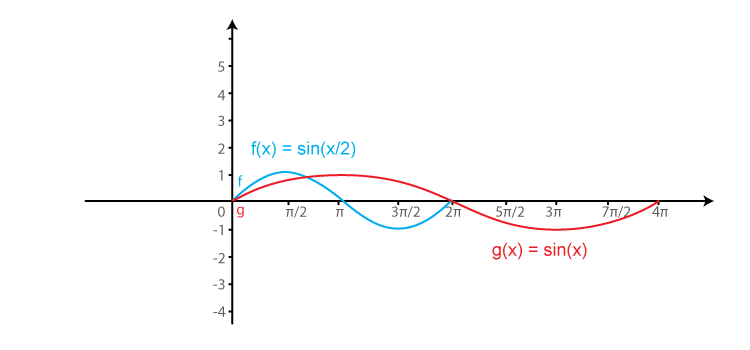# RD Sharma Solutions for Class 11 Chapter 6 - Graphs of Trigonometric Functions Exercise 6.1

In Exercise 6.1 of Chapter 6, we shall discuss problems based on the graph of a sine function. Students who aim to secure high marks in their exams, must and should refer to RD Sharma Class 11 Solutions which are prepared by our expert tutors. Solutions here are made easy to help students crack any type of question by using the shortcut methods developed by the tutors. Solutions are provided in the pdf, students can easily download the pdf of this exercise for free from the links given below.

## Download the Pdf of RD Sharma Solutions for Class 11 Maths Exercise 6.1 Chapter 6 – Graphs of Trigonometric Functions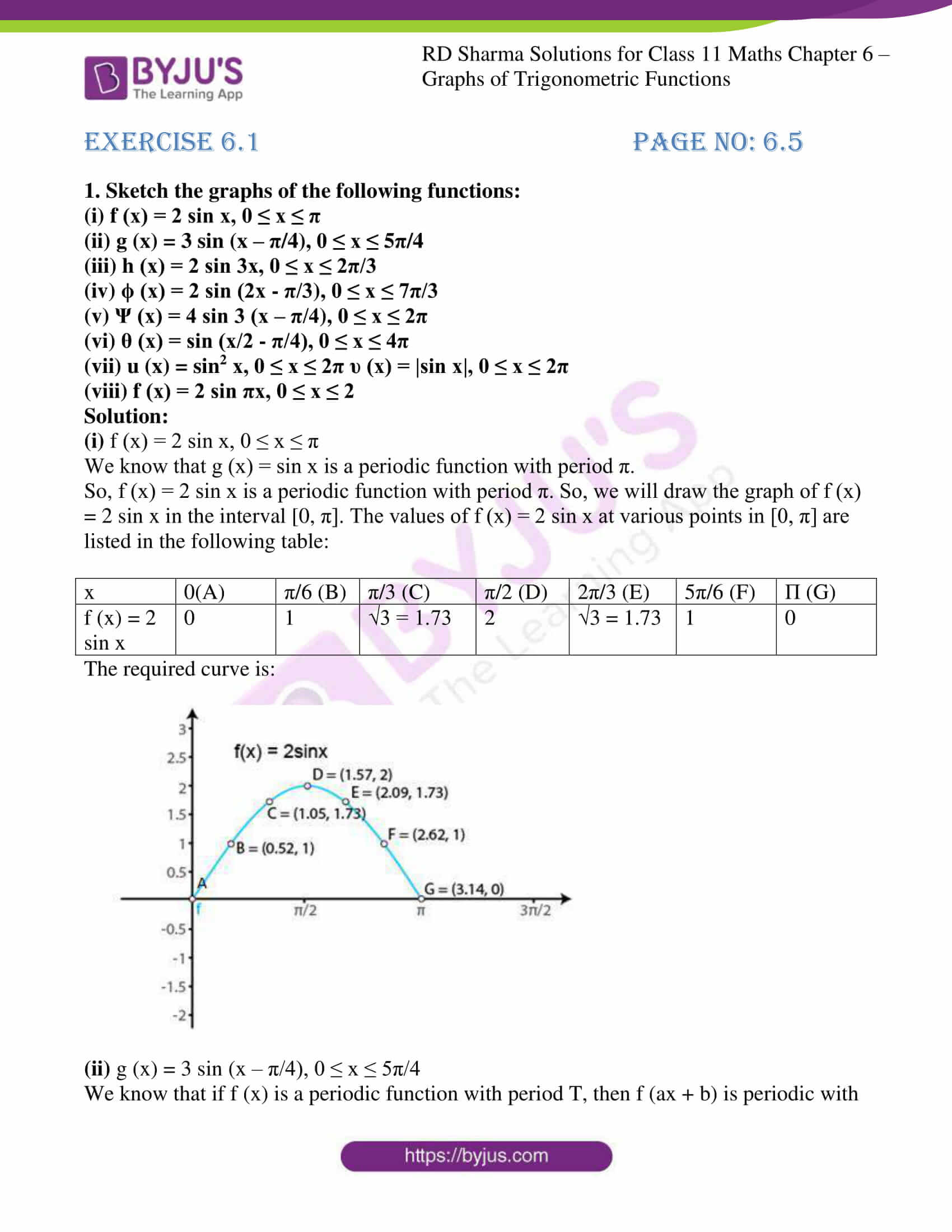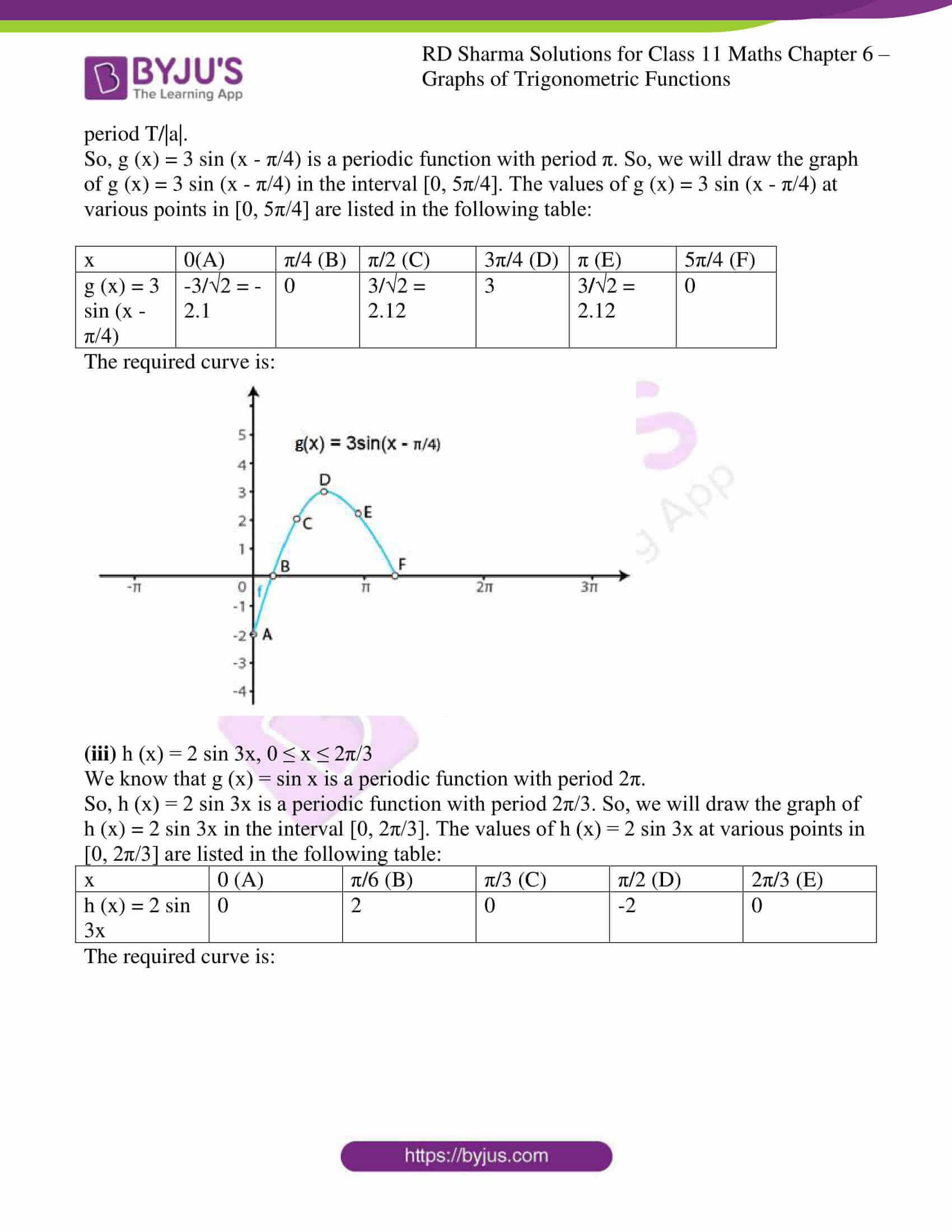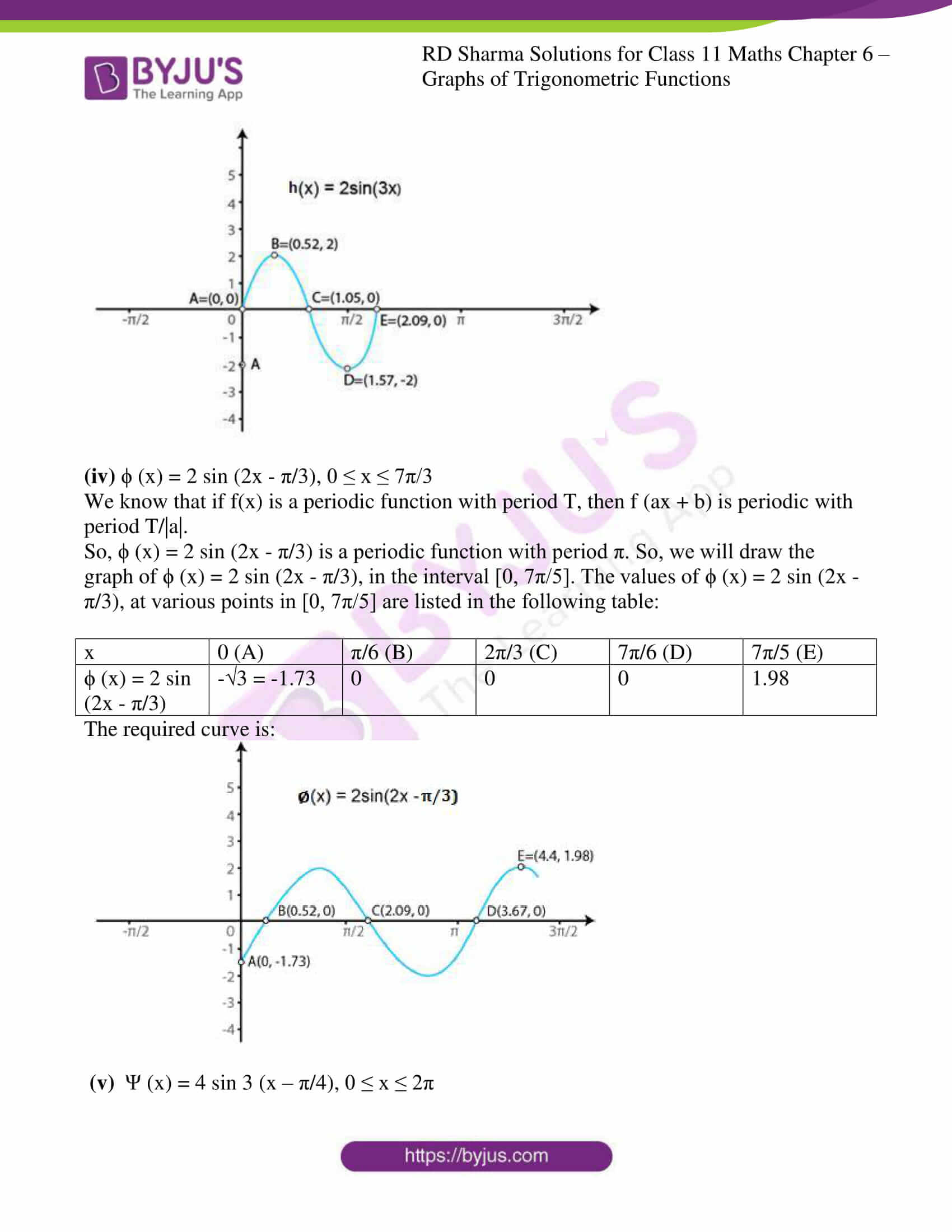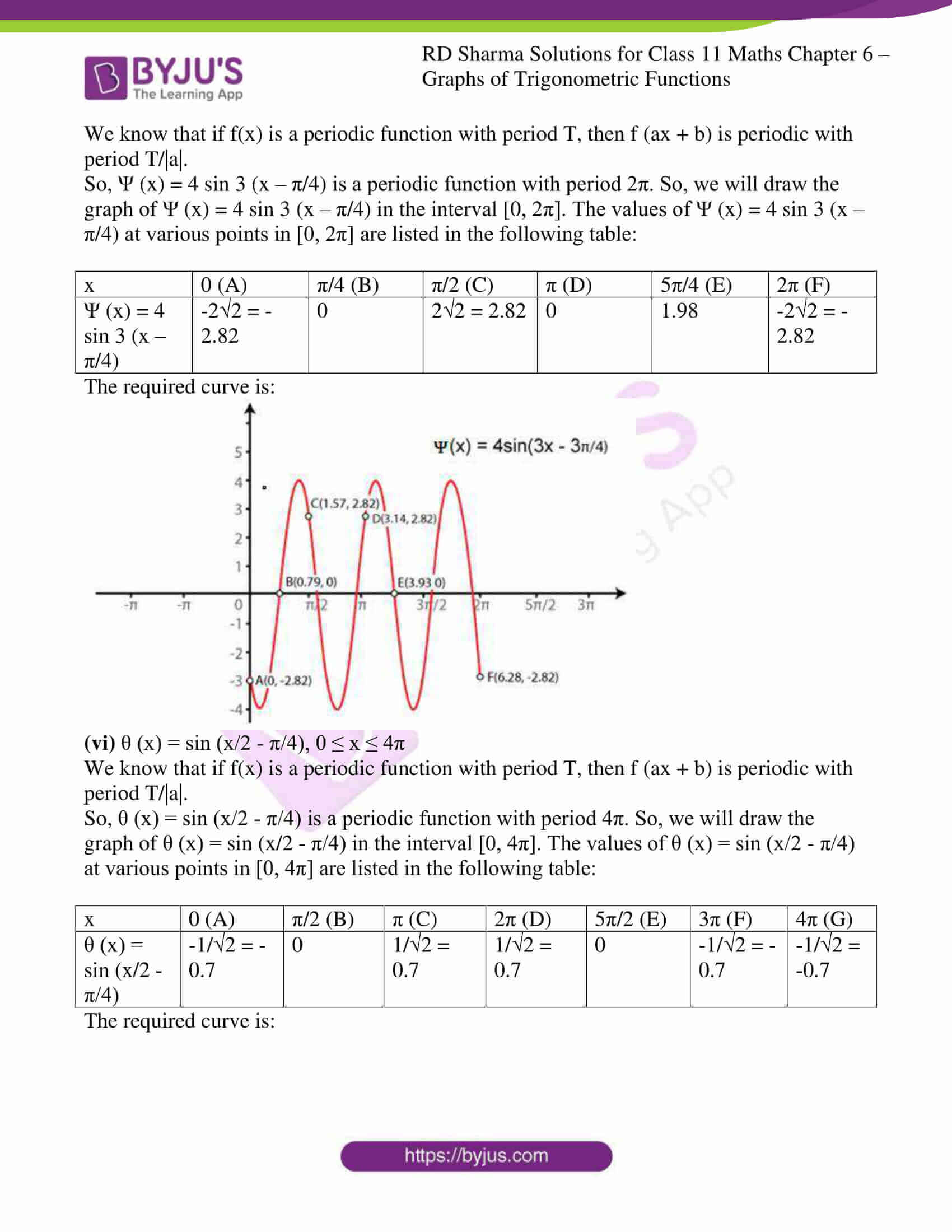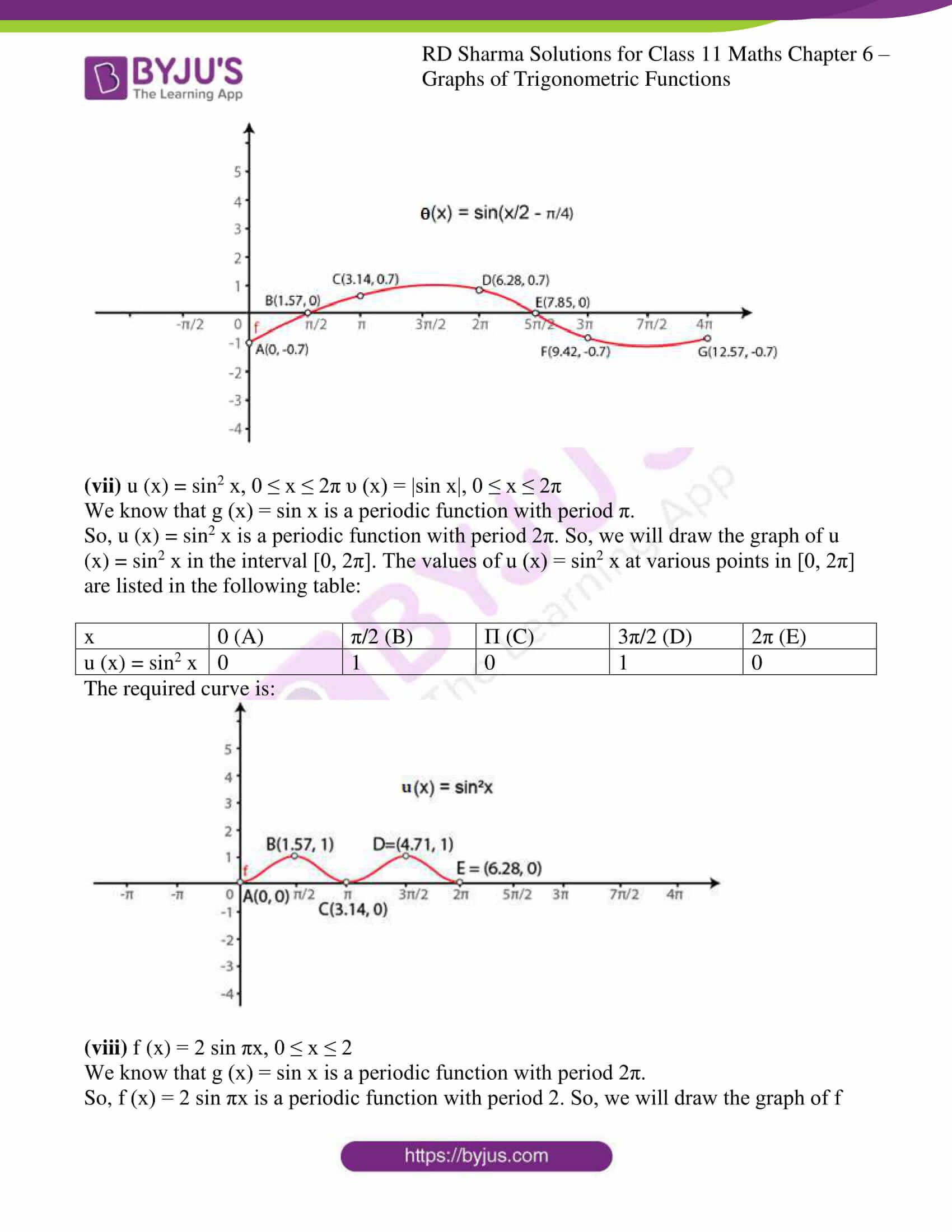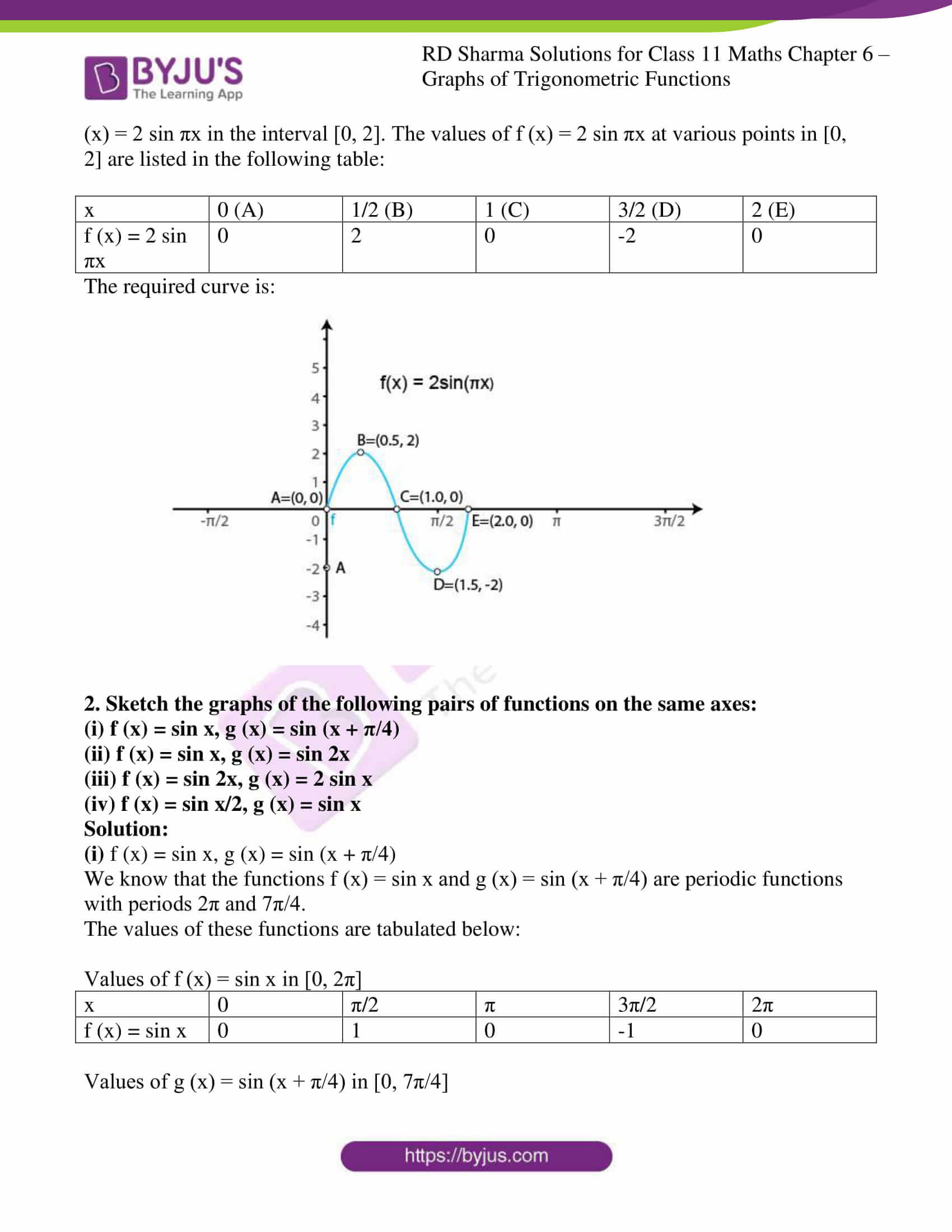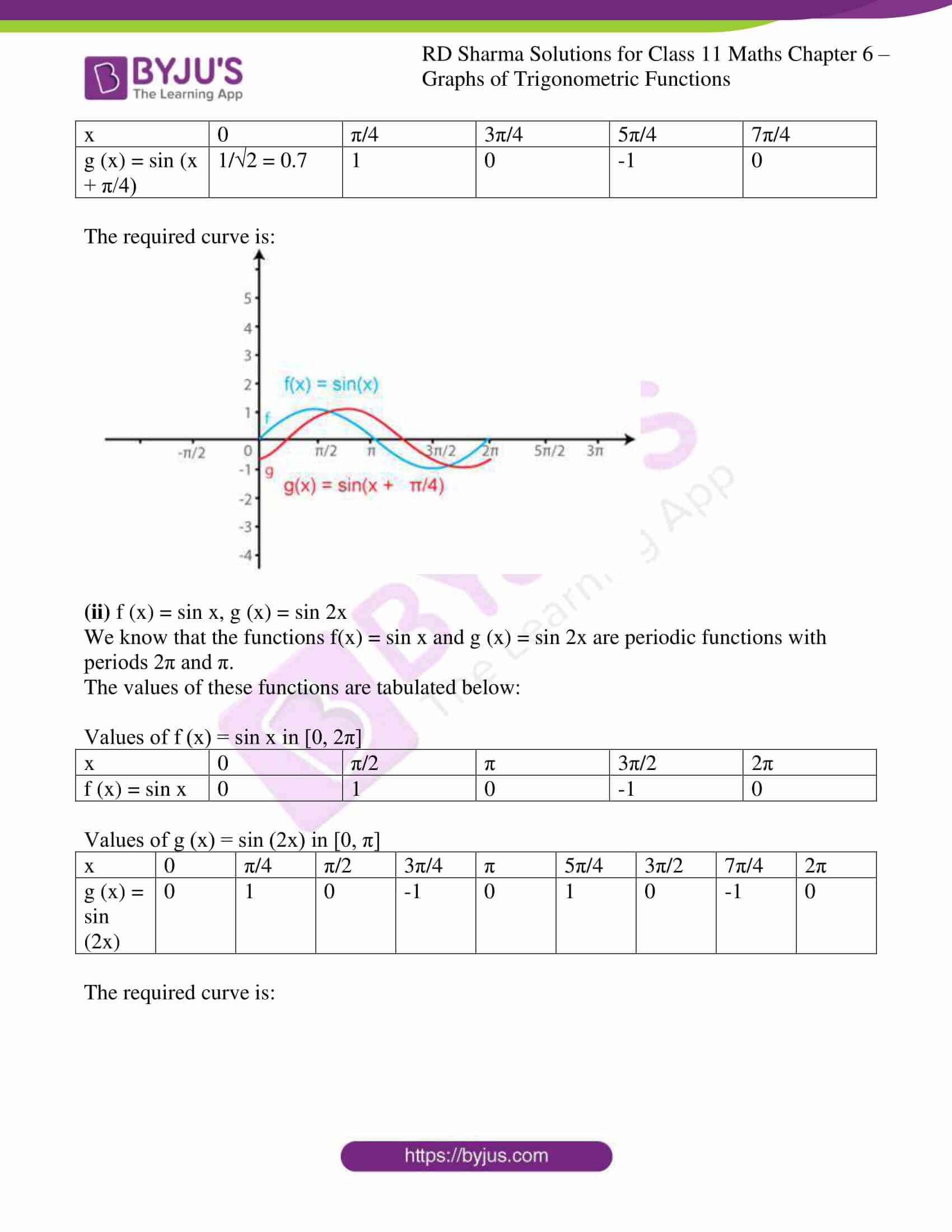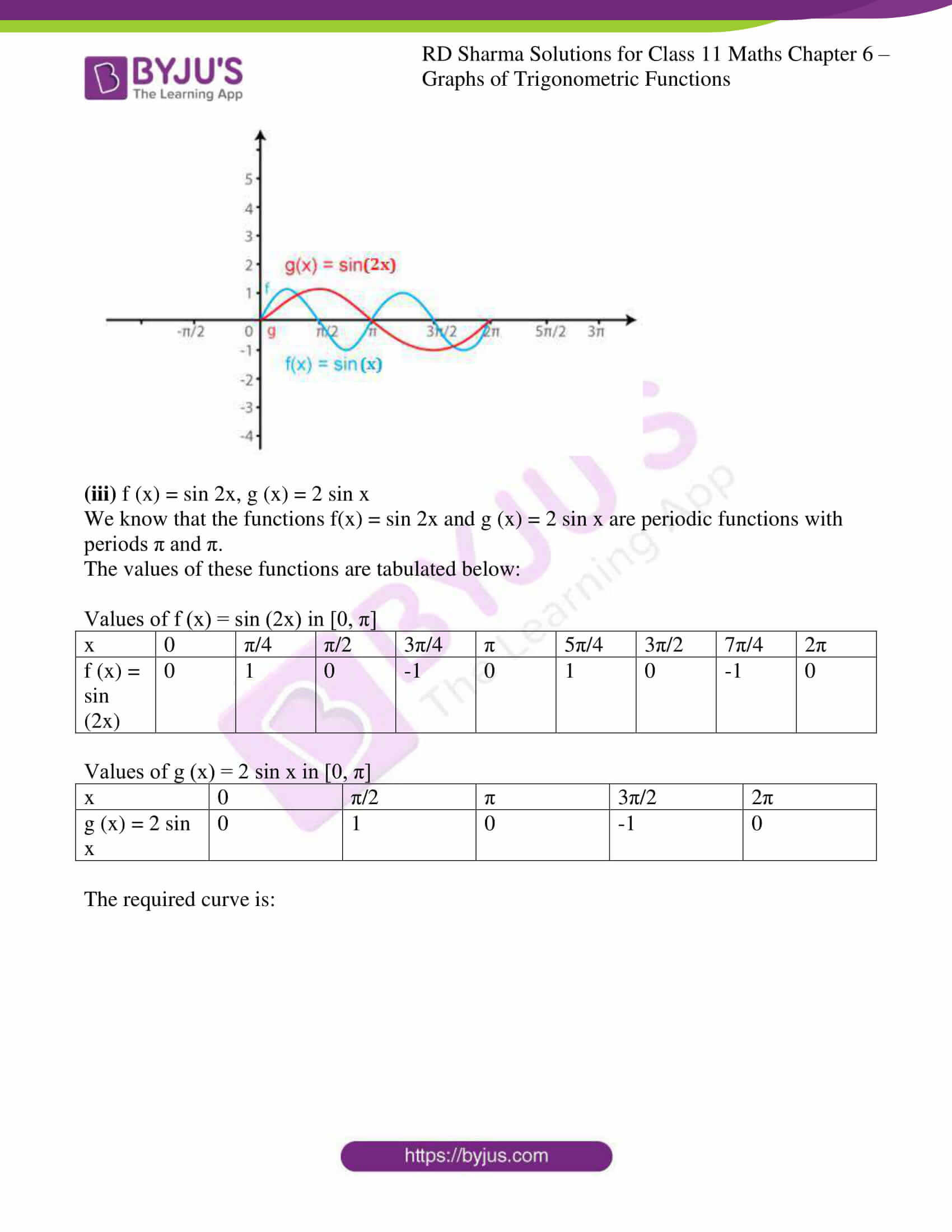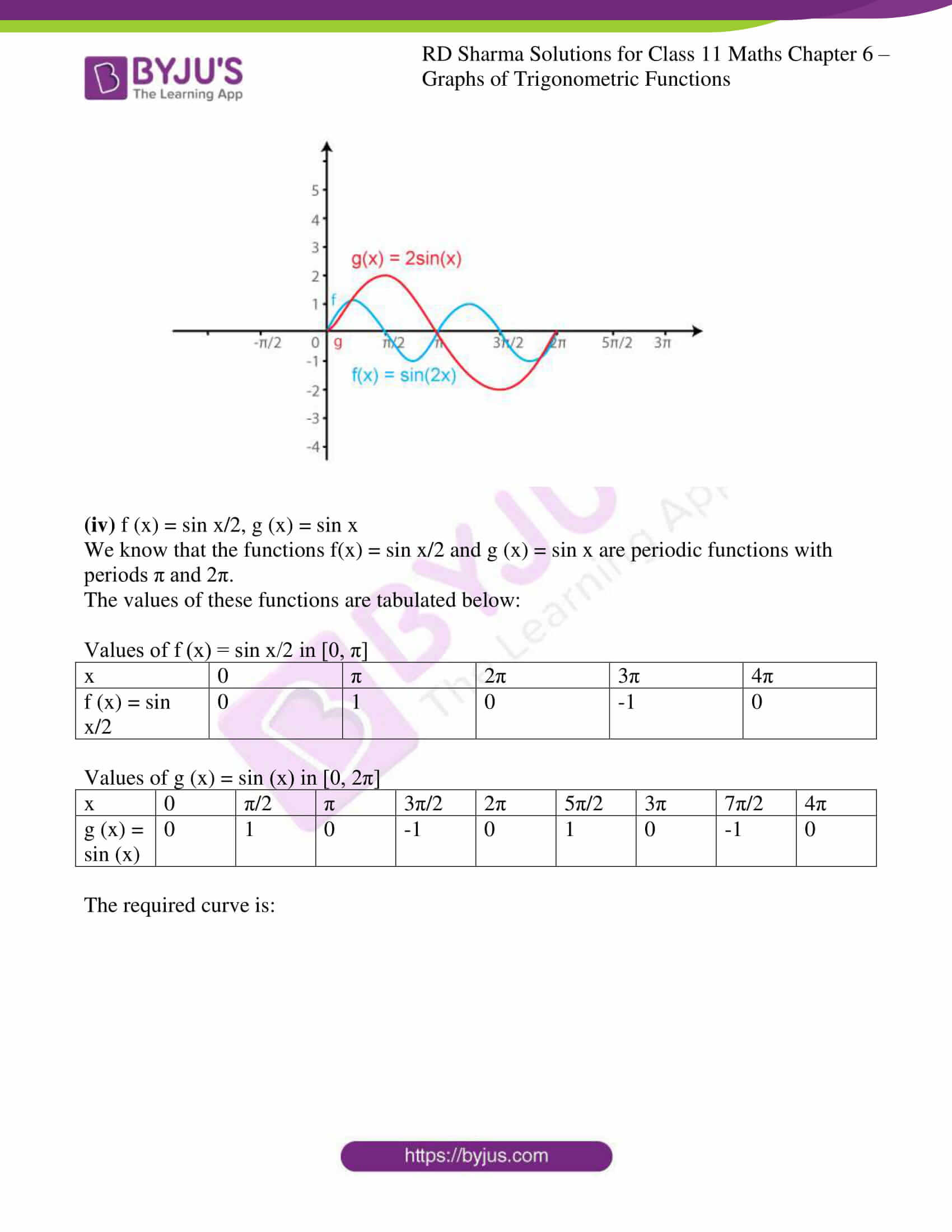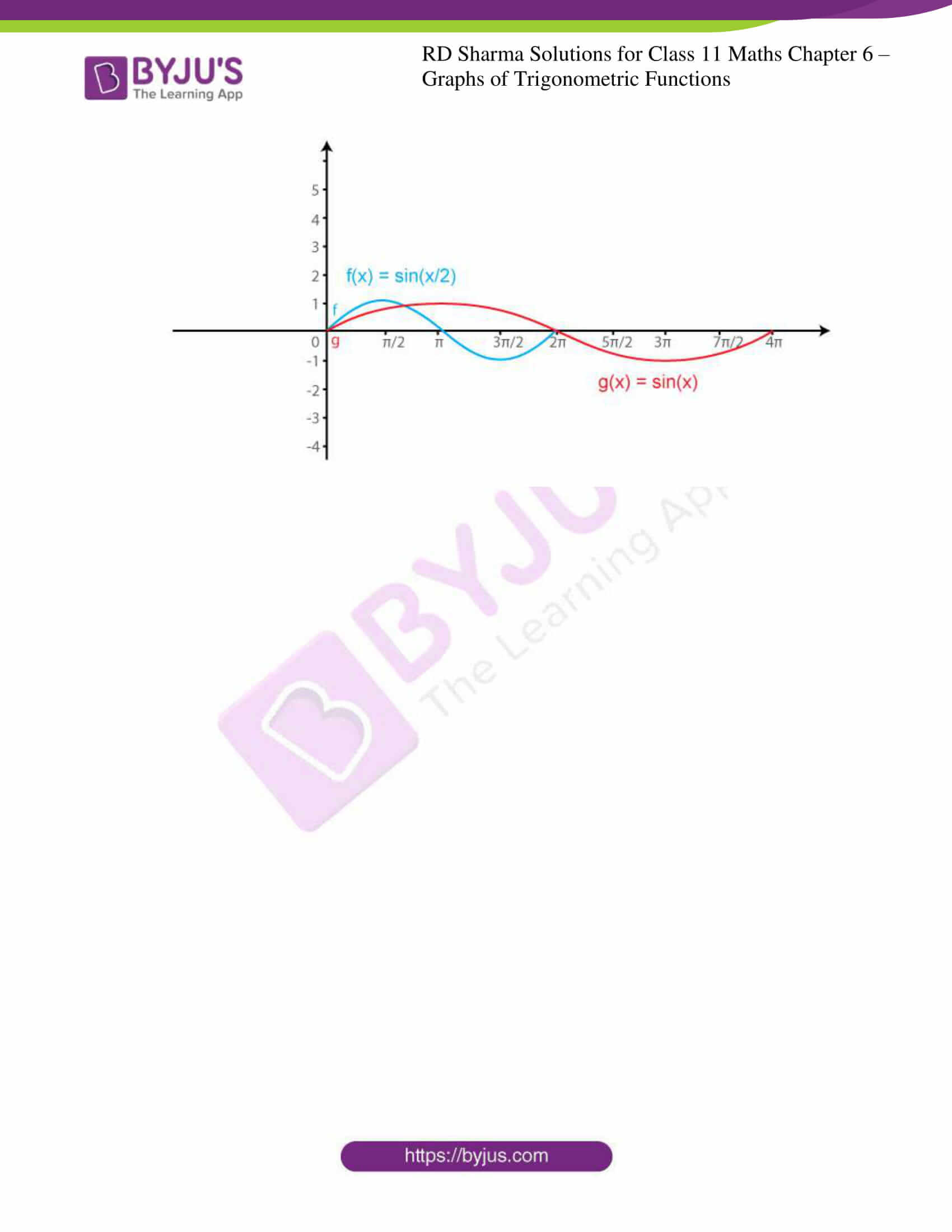### Access answers to RD Sharma Solutions for Class 11 Maths Exercise 6.1 Chapter 6 – Graphs of Trigonometric Functions

1. Sketch the graphs of the following functions:

(i) f (x) = 2 sin x, 0 ≤ x ≤ π

(ii) g (x) = 3 sin (x – π/4), 0 ≤ x ≤ 5π/4

(iii) h (x) = 2 sin 3x, 0 ≤ x ≤ 2π/3

(iv) ϕ (x) = 2 sin (2x – π/3), 0 ≤ x ≤ 7π/3

(v) Ψ (x) = 4 sin 3 (x – π/4), 0 ≤ x ≤ 2π

(vi) θ (x) = sin (x/2 – π/4), 0 ≤ x ≤ 4π

(vii) u (x) = sin2 x, 0 ≤ x ≤ 2π υ (x) = |sin x|, 0 ≤ x ≤ 2π

(viii) f (x) = 2 sin πx, 0 ≤ x ≤ 2

Solution:

(i) f (x) = 2 sin x, 0 ≤ x ≤ π

We know that g (x) = sin x is a periodic function with period π.

So, f (x) = 2 sin x is a periodic function with period π. So, we will draw the graph of f (x) = 2 sin x in the interval [0, π]. The values of f (x) = 2 sin x at various points in [0, π] are listed in the following table:

 x 0(A) π/6 (B) π/3 (C) π/2 (D) 2π/3 (E) 5π/6 (F) Π (G) f (x) = 2 sin x 0 1 √3 = 1.73 2 √3 = 1.73 1 0

The required curve is: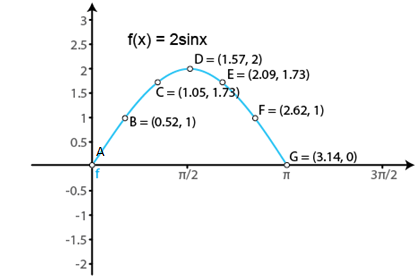(ii) g (x) = 3 sin (x – π/4), 0 ≤ x ≤ 5π/4

We know that if f (x) is a periodic function with period T, then f (ax + b) is periodic with period T/|a|.

So, g (x) = 3 sin (x – π/4) is a periodic function with period π. So, we will draw the graph of g (x) = 3 sin (x – π/4) in the interval [0, 5π/4]. The values of g (x) = 3 sin (x – π/4) at various points in [0, 5π/4] are listed in the following table:

 x 0(A) π/4 (B) π/2 (C) 3π/4 (D) π (E) 5π/4 (F) g (x) = 3 sin (x – π/4) -3/√2 = -2.1 0 3/√2 = 2.12 3 3/√2 = 2.12 0

The required curve is: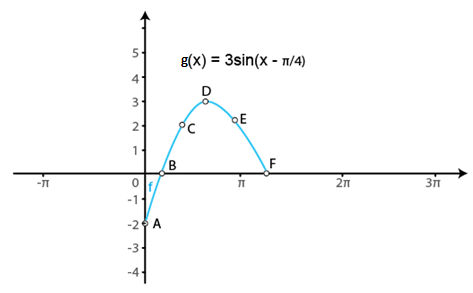(iii) h (x) = 2 sin 3x, 0 ≤ x ≤ 2π/3

We know that g (x) = sin x is a periodic function with period 2π.

So, h (x) = 2 sin 3x is a periodic function with period 2π/3. So, we will draw the graph of h (x) = 2 sin 3x in the interval [0, 2π/3]. The values of h (x) = 2 sin 3x at various points in [0, 2π/3] are listed in the following table:

 x 0 (A) π/6 (B) π/3 (C) π/2 (D) 2π/3 (E) h (x) = 2 sin 3x 0 2 0 -2 0

The required curve is: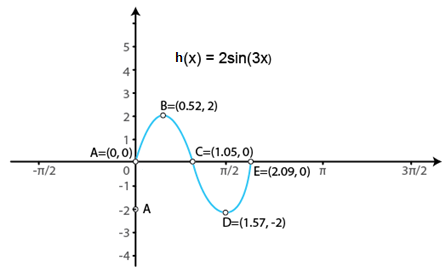(iv) ϕ (x) = 2 sin (2x – π/3), 0 ≤ x ≤ 7π/3

We know that if f(x) is a periodic function with period T, then f (ax + b) is periodic with period T/|a|.

So, ϕ (x) = 2 sin (2x – π/3) is a periodic function with period π. So, we will draw the graph of ϕ (x) = 2 sin (2x – π/3), in the interval [0, 7π/5]. The values of ϕ (x) = 2 sin (2x – π/3), at various points in [0, 7π/5] are listed in the following table:

 x 0 (A) π/6 (B) 2π/3 (C) 7π/6 (D) 7π/5 (E) ϕ (x) = 2 sin (2x – π/3) -√3 = -1.73 0 0 0 1.98

The required curve is: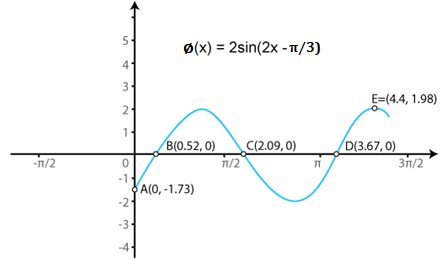(v) Ψ (x) = 4 sin 3 (x – π/4), 0 ≤ x ≤ 2π

We know that if f(x) is a periodic function with period T, then f (ax + b) is periodic with period T/|a|.

So, Ψ (x) = 4 sin 3 (x – π/4) is a periodic function with period 2π. So, we will draw the graph of Ψ (x) = 4 sin 3 (x – π/4) in the interval [0, 2π]. The values of Ψ (x) = 4 sin 3 (x – π/4) at various points in [0, 2π] are listed in the following table:

 x 0 (A) π/4 (B) π/2 (C) π (D) 5π/4 (E) 2π (F) Ψ (x) = 4 sin 3 (x – π/4) -2√2 = -2.82 0 2√2 = 2.82 0 1.98 -2√2 = -2.82

The required curve is: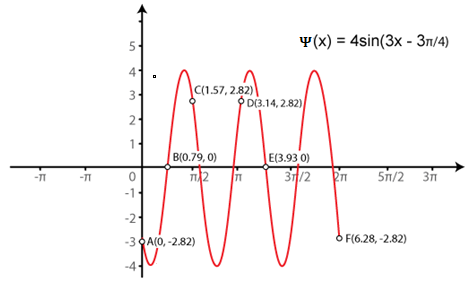(vi) θ (x) = sin (x/2 – π/4), 0 ≤ x ≤ 4π

We know that if f(x) is a periodic function with period T, then f (ax + b) is periodic with period T/|a|.

So, θ (x) = sin (x/2 – π/4) is a periodic function with period 4π. So, we will draw the graph of θ (x) = sin (x/2 – π/4) in the interval [0, 4π]. The values of θ (x) = sin (x/2 – π/4) at various points in [0, 4π] are listed in the following table:

 x 0 (A) π/2 (B) π (C) 2π (D) 5π/2 (E) 3π (F) 4π (G) θ (x) = sin (x/2 – π/4) -1/√2 = -0.7 0 1/√2 = 0.7 1/√2 = 0.7 0 -1/√2 = -0.7 -1/√2 = -0.7

The required curve is: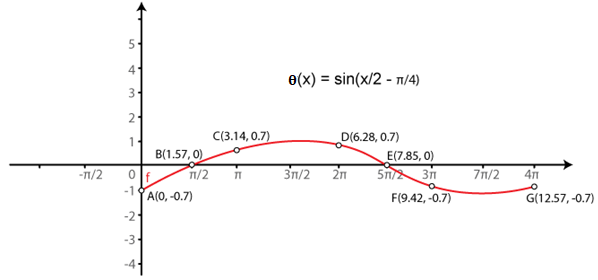(vii) u (x) = sin2 x, 0 ≤ x ≤ 2π υ (x) = |sin x|, 0 ≤ x ≤ 2π

We know that g (x) = sin x is a periodic function with period π.

So, u (x) = sin2 x is a periodic function with period 2π. So, we will draw the graph of u (x) = sin2 x in the interval [0, 2π]. The values of u (x) = sin2 x at various points in [0, 2π] are listed in the following table:

 x 0 (A) π/2 (B) Π (C) 3π/2 (D) 2π (E) u (x) = sin2 x 0 1 0 1 0

The required curve is: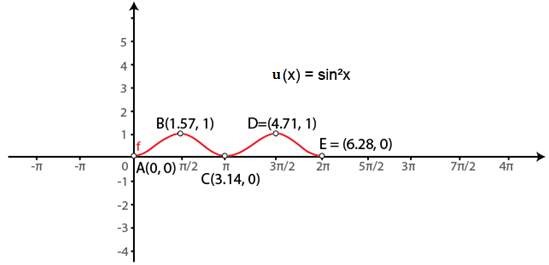(viii) f (x) = 2 sin πx, 0 ≤ x ≤ 2

We know that g (x) = sin x is a periodic function with period 2π.

So, f (x) = 2 sin πx is a periodic function with period 2. So, we will draw the graph of f (x) = 2 sin πx in the interval [0, 2]. The values of f (x) = 2 sin πx at various points in [0, 2] are listed in the following table:

 x 0 (A) 1/2 (B) 1 (C) 3/2 (D) 2 (E) f (x) = 2 sin πx 0 2 0 -2 0

The required curve is: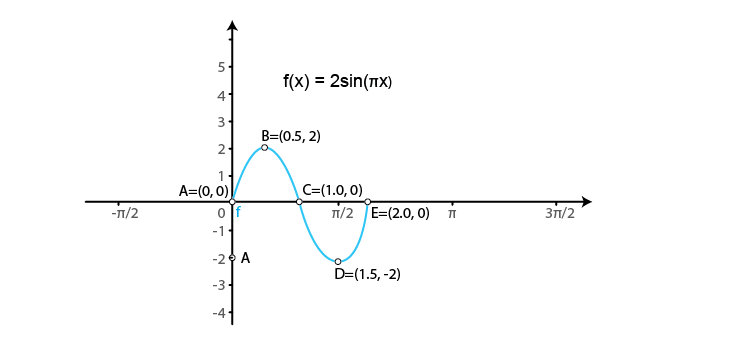2. Sketch the graphs of the following pairs of functions on the same axes:

(i) f (x) = sin x, g (x) = sin (x + π/4)

(ii) f (x) = sin x, g (x) = sin 2x

(iii) f (x) = sin 2x, g (x) = 2 sin x

(iv) f (x) = sin x/2, g (x) = sin x

Solution:

(i) f (x) = sin x, g (x) = sin (x + π/4)

We know that the functions f (x) = sin x and g (x) = sin (x + π/4) are periodic functions with periods 2π and 7π/4.

The values of these functions are tabulated below:

Values of f (x) = sin x in [0, 2π]

 x 0 π/2 π 3π/2 2π f (x) = sin x 0 1 0 -1 0

Values of g (x) = sin (x + π/4) in [0, 7π/4]

 x 0 π/4 3π/4 5π/4 7π/4 g (x) = sin (x + π/4) 1/√2 = 0.7 1 0 -1 0

The required curve is: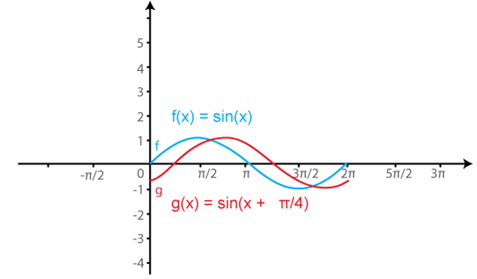(ii) f (x) = sin x, g (x) = sin 2x

We know that the functions f(x) = sin x and g (x) = sin 2x are periodic functions with periods 2π and π.

The values of these functions are tabulated below:

Values of f (x) = sin x in [0, 2π]

 x 0 π/2 π 3π/2 2π f (x) = sin x 0 1 0 -1 0

Values of g (x) = sin (2x) in [0, π]

 x 0 π/4 π/2 3π/4 π 5π/4 3π/2 7π/4 2π g (x) = sin (2x) 0 1 0 -1 0 1 0 -1 0

The required curve is: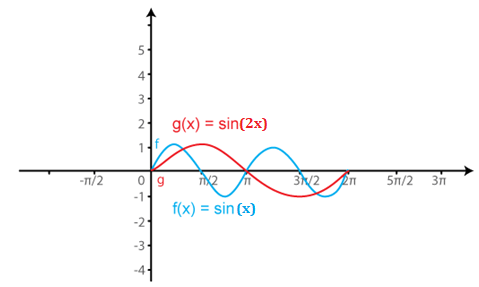(iii) f (x) = sin 2x, g (x) = 2 sin x

We know that the functions f(x) = sin 2x and g (x) = 2 sin x are periodic functions with periods π and π.

The values of these functions are tabulated below:

Values of f (x) = sin (2x) in [0, π]

 x 0 π/4 π/2 3π/4 π 5π/4 3π/2 7π/4 2π f (x) = sin (2x) 0 1 0 -1 0 1 0 -1 0

Values of g (x) = 2 sin x in [0, π]

 x 0 π/2 π 3π/2 2π g (x) = 2 sin x 0 1 0 -1 0

The required curve is: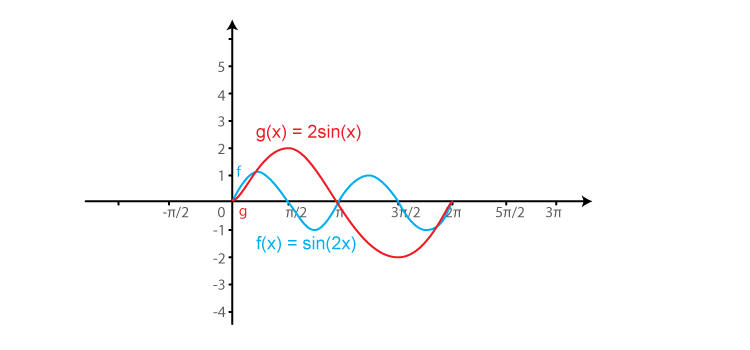(iv) f (x) = sin x/2, g (x) = sin x

We know that the functions f(x) = sin x/2 and g (x) = sin x are periodic functions with periods π and 2π.

The values of these functions are tabulated below:

Values of f (x) = sin x/2 in [0, π]

 x 0 π 2π 3π 4π f (x) = sin x/2 0 1 0 -1 0

Values of g (x) = sin (x) in [0, 2π]

 x 0 π/2 π 3π/2 2π 5π/2 3π 7π/2 4π g (x) = sin (x) 0 1 0 -1 0 1 0 -1 0

The required curve is: